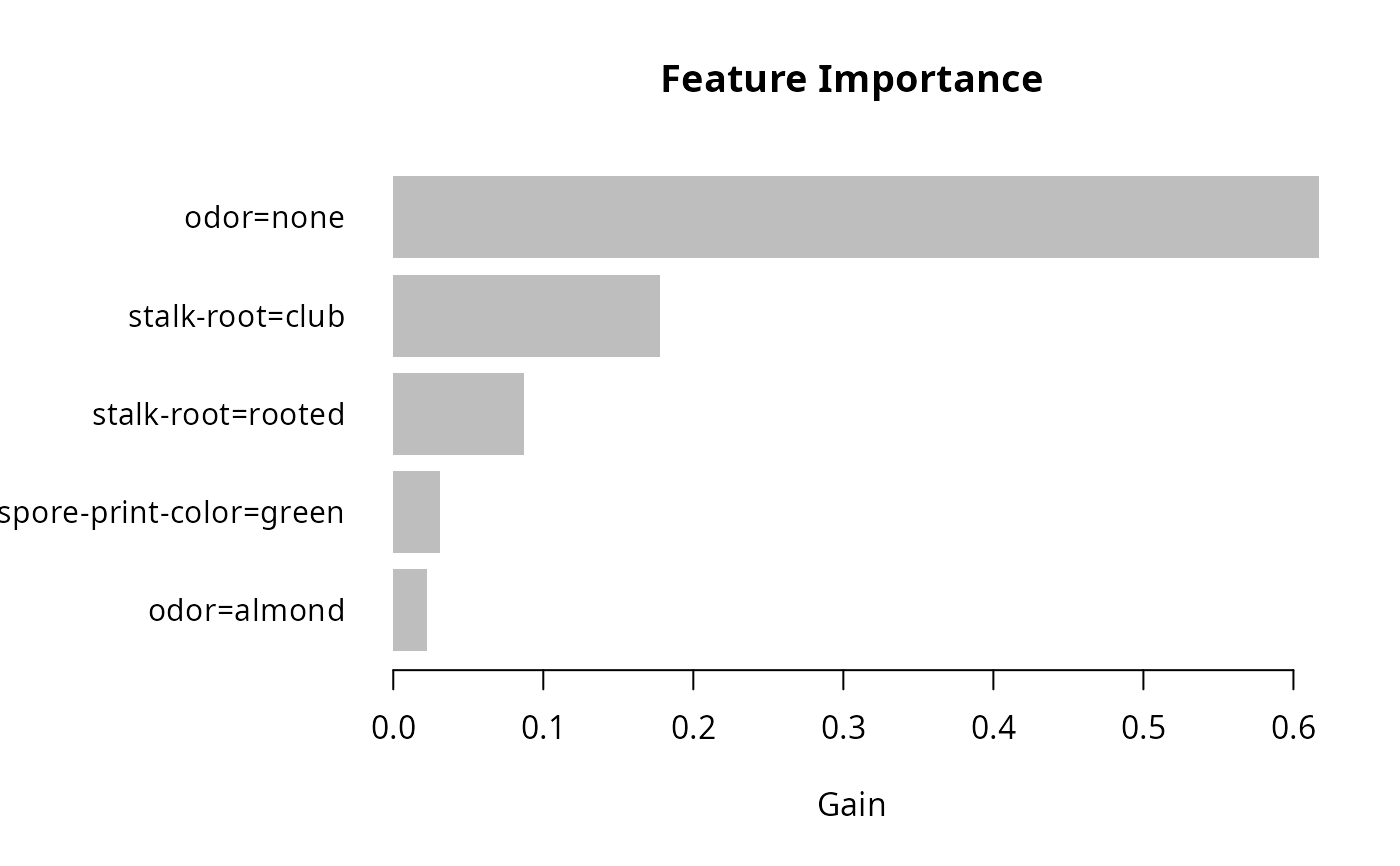Plot previously calculated feature importance: Gain, Cover and Frequency, as a bar graph.

lgb.plot.importance(
tree_imp,
top_n = 10L,
measure = "Gain",
left_margin = 10L,
cex = NULL
)

## Arguments

tree_imp a data.table returned by lgb.importance. maximal number of top features to include into the plot. the name of importance measure to plot, can be "Gain", "Cover" or "Frequency". (base R barplot) allows to adjust the left margin size to fit feature names. (base R barplot) passed as cex.names parameter to barplot. Set a number smaller than 1.0 to make the bar labels smaller than R's default and values greater than 1.0 to make them larger.

## Value

The lgb.plot.importance function creates a barplot and silently returns a processed data.table with top_n features sorted by defined importance.

## Details

The graph represents each feature as a horizontal bar of length proportional to the defined importance of a feature. Features are shown ranked in a decreasing importance order.

## Examples

# \donttest{
data(agaricus.train, package = "lightgbm")
train <- agaricus.train
dtrain <- lgb.Dataset(train$data, label = train$label)

params <- list(
objective = "binary"
, learning_rate = 0.1
, min_data_in_leaf = 1L
, min_sum_hessian_in_leaf = 1.0
)

model <- lgb.train(
params = params
, data = dtrain
, nrounds = 5L
)
#> [LightGBM] [Info] Number of positive: 3140, number of negative: 3373
#> [LightGBM] [Info] Auto-choosing row-wise multi-threading, the overhead of testing was 0.000937 seconds.
#> You can set force_row_wise=true to remove the overhead.
#> And if memory is not enough, you can set force_col_wise=true.
#> [LightGBM] [Info] Total Bins 232
#> [LightGBM] [Info] Number of data points in the train set: 6513, number of used features: 116
#> [LightGBM] [Info] [binary:BoostFromScore]: pavg=0.482113 -> initscore=-0.071580
#> [LightGBM] [Info] Start training from score -0.071580
#> [LightGBM] [Warning] No further splits with positive gain, best gain: -inf
#> [LightGBM] [Warning] No further splits with positive gain, best gain: -inf
#> [LightGBM] [Warning] No further splits with positive gain, best gain: -inf
#> [LightGBM] [Warning] No further splits with positive gain, best gain: -inf
#> [LightGBM] [Warning] No further splits with positive gain, best gain: -inf

tree_imp <- lgb.importance(model, percentage = TRUE)
lgb.plot.importance(tree_imp, top_n = 5L, measure = "Gain")# }